Question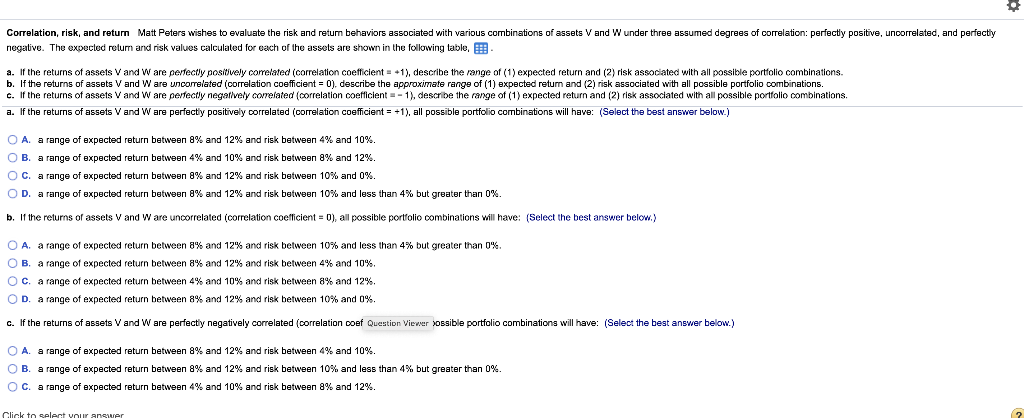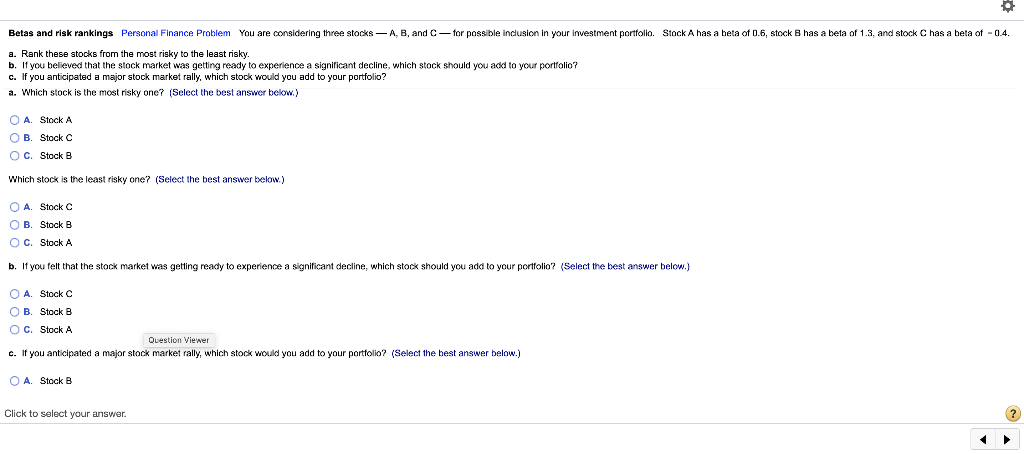a.

The most risky one

C.stock B.

The one with high beta is the most risky one.

Least risky one.

A. stock C.

The one with low beta is the least risky one.

b.

A.Stock C.

If market is going to decline the one with negative beta can be added to portfolio.

c.

A.stock B.

If a rally is anticipated, the one with high beta can be added.

#### Earn Coins

Coins can be redeemed for fabulous gifts.

Similar Homework Help Questions
• ### LG4 5-14 Correlation, risk, and return Matt Peters wishes to evaluate the risk and return behaviors...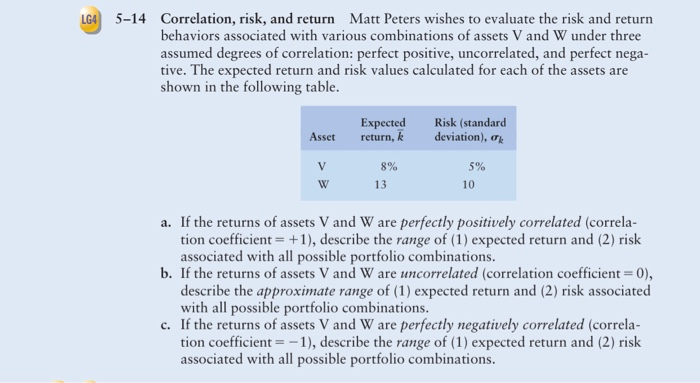LG4 5-14 Correlation, risk, and return Matt Peters wishes to evaluate the risk and return behaviors associated with various combinations of assets V and W under three assumed degrees of correlation: perfect positive, uncorrelated, and perfect nega- tive. The expected return and risk values calculated for each of the assets are shown in the following table. Asset Expected return, k Risk (standard deviation), V 5% 8% 13 a. If the returns of assets V and W are perfectly positively correlated...

• ### Assume you wish to evaluate the risk and return behaviors associated with various combinations of assets...

Assume you wish to evaluate the risk and return behaviors associated with various combinations of assets V and W under three assumed degrees of​ correlation: perfect​ positive, uncorrelated, and perfect negative. The following average return and risk values were calculated for these​ assets: Asset    Average​ Return, r Risk​ (Standard Deviation), s V 7.9​% 4.6​% W 12.7​% 9.7​% a. If the returns of assets V and W are perfectly positively correlated​ (correlation coefficient = + 1​), describe the range of​...

• ### Assume you wish to evaluate the risk and return behaviors associated with various combinations of two​ stocks, Alpha Sof...

Assume you wish to evaluate the risk and return behaviors associated with various combinations of two​ stocks, Alpha Software and Beta​ Electronics, under three possible degrees of​ correlation: perfect​ positive, uncorrelated, and perfect negative. The average return and standard deviation for each stock appears​ here: Asset   Average Return,overbar r                   Risk (Standard Deviation), s Alpha   5.1%   30.3% Beta                    11.2%      50.5% a. If the returns of assets Alpha and Beta are perfectly positively correlated​ (correlation coefficient equals plus 1​),...

• ### le three alternatives. c. Use your findings in parts a and b to calculate the coefficient of variatio each of the three...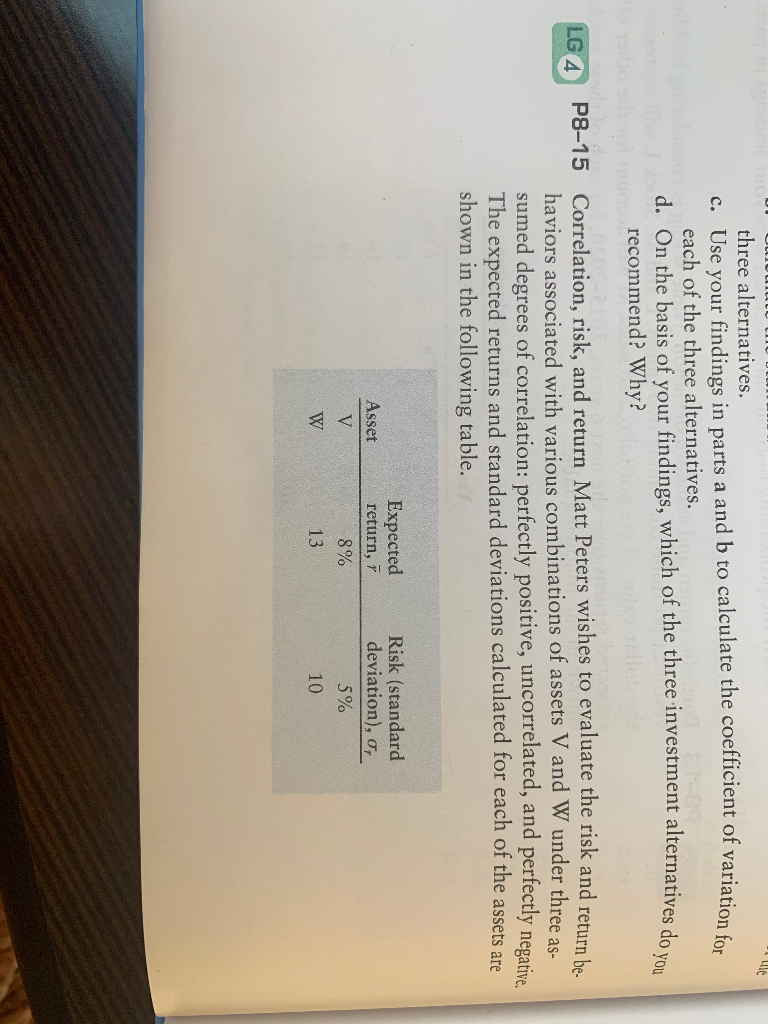le three alternatives. c. Use your findings in parts a and b to calculate the coefficient of variatio each of the three alternatives. d. On the basis of your findings, which of the three investment alternatives do recommend? Why? LG 4 P8-15 Correlation, risk, and return Matt Peters wishes to evaluate the risk and return be haviors associated with various combinations of assets V and W under three as- sumed degrees of correlation: perfectly positive, uncorrelated, and perfectly negative The...

• ### p8-15 A-C 333 CHAPTER 8 Risk and Return a. If the returns of assets V and...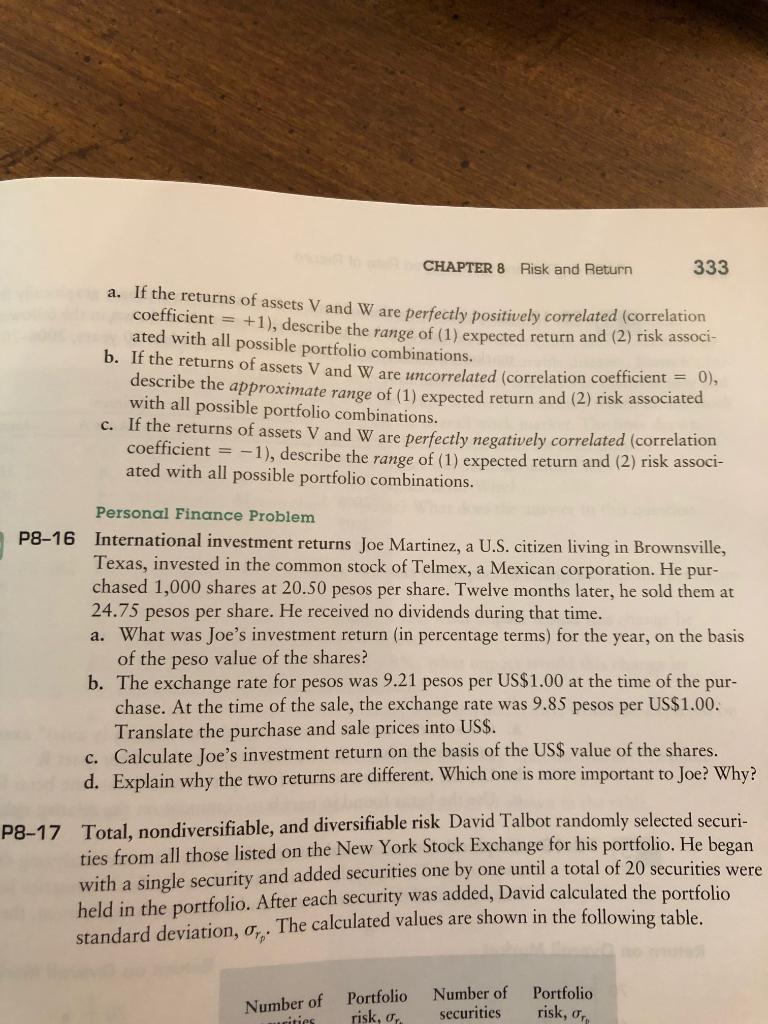p8-15 A-C 333 CHAPTER 8 Risk and Return a. If the returns of assets V and W are perfectly positively correlated (correlation coefficient = +1), describe the range of (1) expected return and (2) risk associ- ated with all possible portfolio combinations. b. If the returns of assets V and W are uncorrelated (correlation coefficient = 0), describe the approximate range of (1) expected return and (2) risk associated with all possible portfolio combinations c. If the returns of assets...

• ### 2. 3: Risk and Rates of Return: Risk in Portfolio Context Risk and Rates of Return:...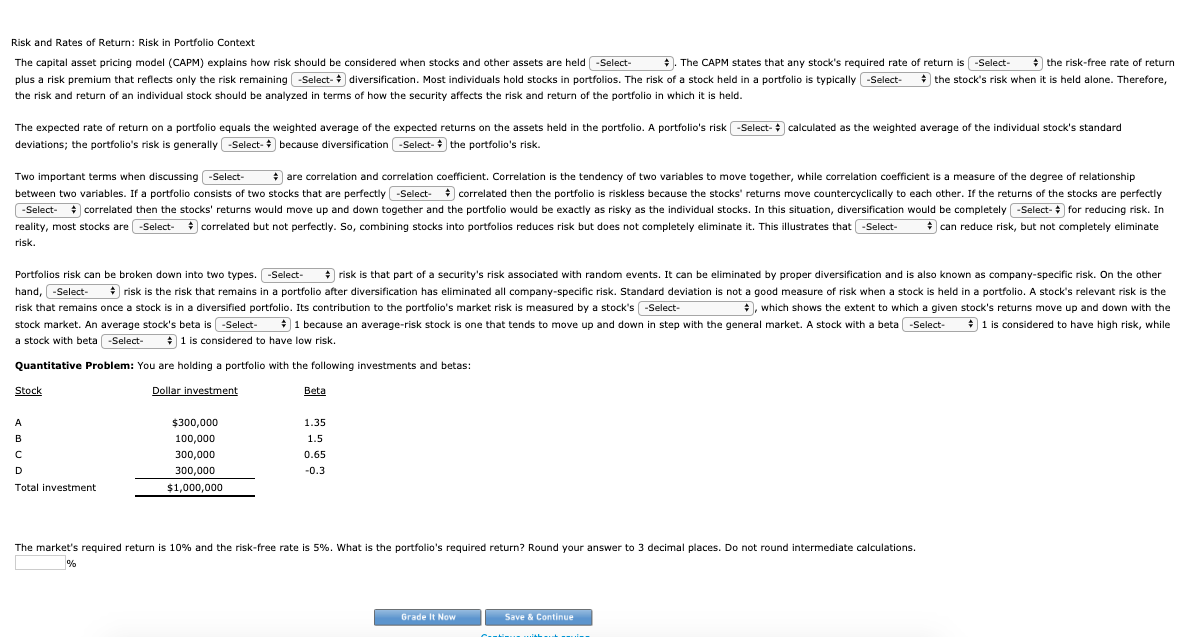2. 3: Risk and Rates of Return: Risk in Portfolio Context Risk and Rates of Return: Risk in Portfolio Context The capital asset pricing model (CAPM) explains how risk should be considered when stocks and other assets are held . The CAPM states that any stock's required rate of return is the risk-free rate of return plus a risk premium that reflects only the risk remaining diversification. Most individuals hold stocks in portfolios. The risk of a stock held in...

• ### A correlation coefficient of .068 would indicate that

A correlation coefficient of .068 would indicate thata 6.8% return would be earned with the investmentthe returns are virtually uncorrelatedthe risk of the investment portfolio is almost zerothe returns are strongly (almost perfectly) positively correlated

• ### od The capital asset pricing model (CAPM) explains how risk should be considered when stocks and...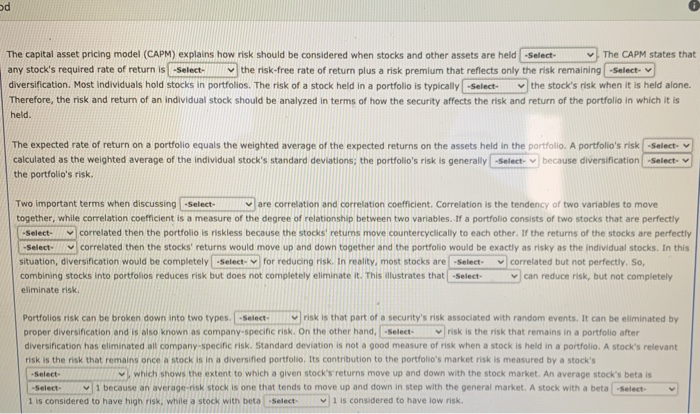od The capital asset pricing model (CAPM) explains how risk should be considered when stocks and other assets are held -Select- The CAPM states that any stock's required rate of return is -Select the risk-free rate of return plus a risk premium that reflects only the risk remaining -Select- diversification. Most individuals hold stocks in portfolios. The risk of a stock held in a portfolio is typically -Select the stock's risk when it is held alone. Therefore, the risk and...

• ### (a) Suppose that there are many stocks in the security market and that the characteristics of...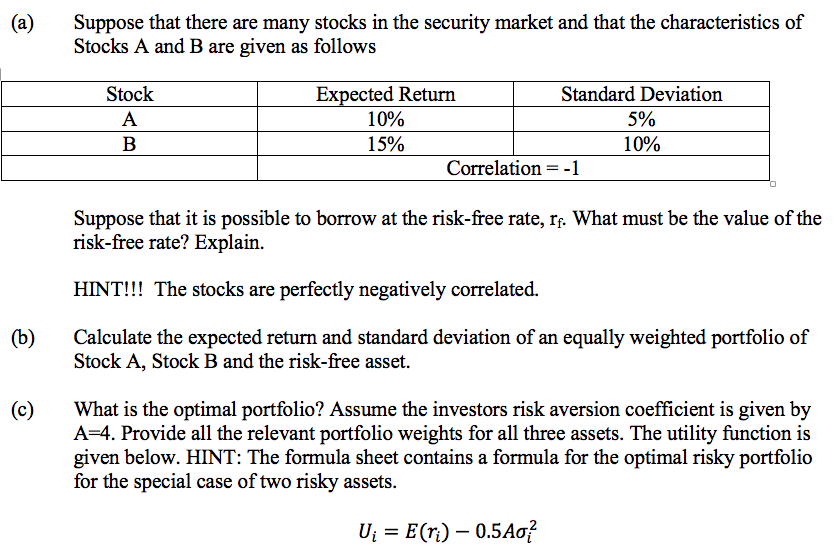(a) Suppose that there are many stocks in the security market and that the characteristics of Stocks A and B are given as follows Stock A B Expected Return Standard Deviation 10% 5% 15% 10% Correlation =-1 Suppose that it is possible to borrow at the risk-free rate, If. What must be the value of the risk-free rate? Explain. HINT!!! The stocks are perfectly negatively correlated. (b) Calculate the expected return and standard deviation of an equally weighted portfolio of...

• ### Term Answer Description Risk A. The potential for variability in the possible outcomes associated with an...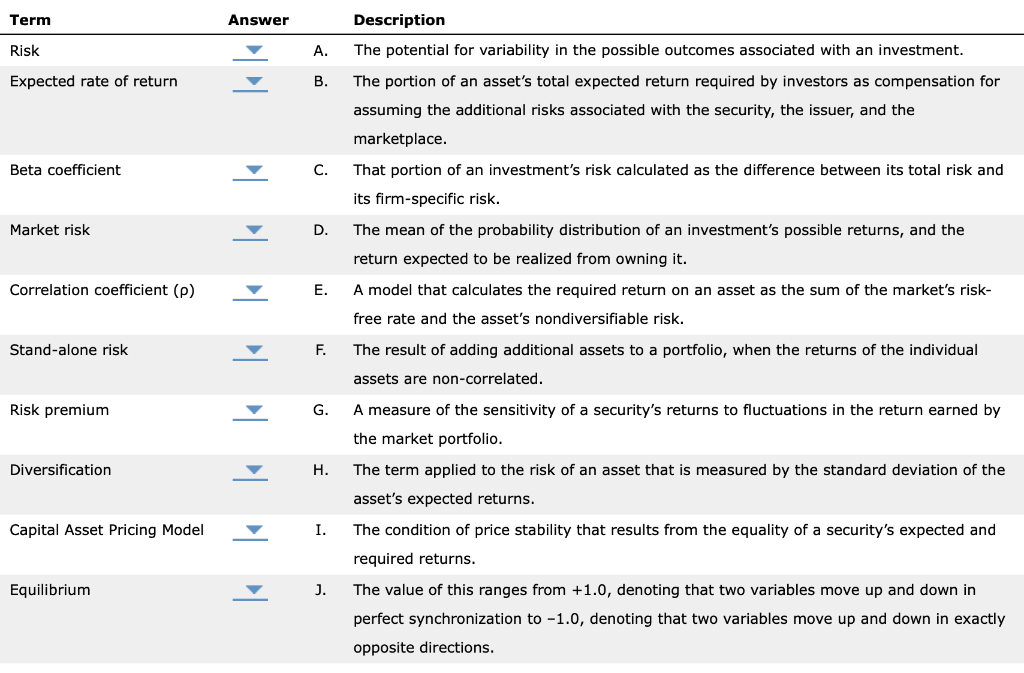Term Answer Description Risk A. The potential for variability in the possible outcomes associated with an investment. Expected rate of return The portion of an asset's total expected return required by investors as compensation for assuming the additional risks associated with the security, the issuer, and the marketplace. Beta coefficient That portion of an investment's risk calculated as the difference between its total risk and its firm-specific risk. Market risk The mean of the probability distribution of an investment's possible...